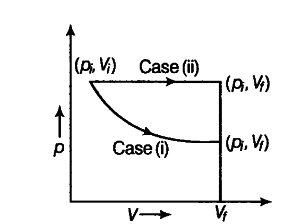# Plot the p-V diagram for each case

The initial state of a certain gas is ({ p }_{ i },
{ v }_{ i },
{ t }_{ i } ). It undergoes expansion till its volume becomes
{ V }_{ f }. Consider the following two cases
(i) The expansion takes place at constant temperature.
(ii) The expansion takes place at constant pressure.
Plot the p-V diagram for each case. In which of the two cases, is the work done by the gas more?The p-Vdiagram for each case is shown in the figure.
In case(i) piVi = pfVf , therefore process is isothermal. In case(ii) work done = area under the p-v curve so work done is more when the gas expands at constant pressure.